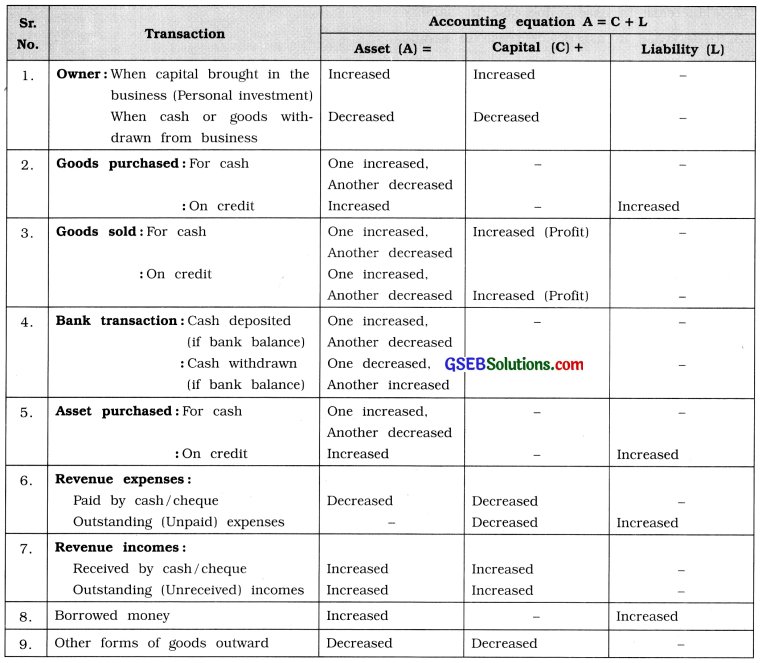# GSEB Class 11 Accounts Notes Part 1 Chapter 5 Accounting Equation and Business Transactions

This GSEB Class 11 Commerce Accounts Notes Part 1 Chapter 5 Accounting Equation and Business Transactions covers all the important topics and concepts as mentioned in the chapter.

## Accounting Equation and Business Transactions Class 11 GSEB Notes

Accounting transaction affects into at least two accounts. One account receives consideration or gain, the other account gives consideration or sacrifies the gain. And therefore, as a result of certain transactions, changes in assets and in liabilities are observed.

If we put this thing in formula we get the following results :
A = C + L.
Where, A = Assets;
C = Capital and
L = Liabilities.

This is also known as Accounting Equation. Increase or decrease in one asset, may be because of the opposite effect of changes in some other asset, in such circumstances total assets of the business remains unchanged. But when assets are purchased on credit, on one side assets increases and on the other side liability also increases with an equal amount.

When new capital investment is brought in the business, capital as well as assets are also increases. Capital is also effected by the transactions of purchase and sale of goods and by the transactions of incomes and expenses, under Double Entry System of book-keeping. Every business transaction has two-fold effects on business entity and the dual aspect of each transaction affect changes in assets, liabilities and capital in such a manner that the basic accounting equation (A = C + L) is satisfied. If we examine two-fold effects of any transaction, it results into increase/decrease in assets and/or increase / decrease in liability or capital.

→ To know the business result, at the end of the year final accounts are prepared. In which Trading Account, Profit and Loss Account and Balance Sheet are prepared.

→ At the end of the year Profit and Loss Account will be closed and it will be transferred to Balance Sheet.

→ There are two important components of Balance Sheet:(1) Capital & Liability and (2) Assets.

→ Assets = Capital + Liabilities means A = C + L.→ Always every transaction has dual effects, which is effected on A = C + L is in this manner :

• If A increased then C or L also increased, therefore A = C + L is maintained.
• If A decreased then C or L also decreased, therefore A = C + L is maintained.
• A increased-A decreased, opposite effect is in only one side, therefore A = C + L is maintained.
• Increased and decreased in C or in L only, therefore A = C + L is maintained.

→ Capital decreased due to expenses and Capital increased due to incomes.